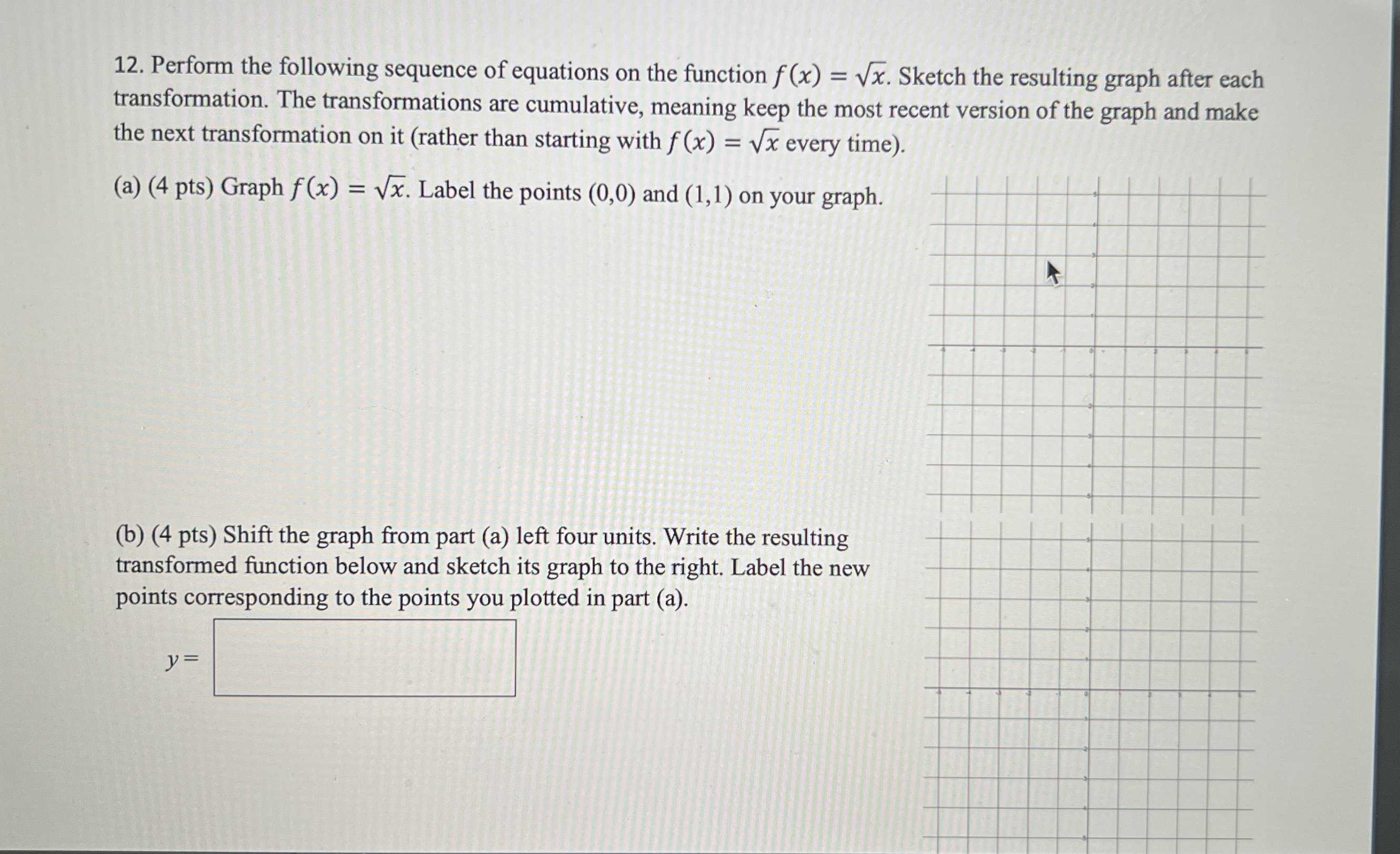### ¿Todavía tienes preguntas de matemáticas?

Pregunte a nuestros tutores expertos
Algebra
Pregunta12. Perform the following sequence of equations on the function $$f ( x ) = \sqrt { x }$$ . Sketch the resulting graph after each transformation. The transformations are cumulative, meaning keep the most recent version of the graph and make the next transformation on it (rather than starting with $$f ( x ) = \sqrt { x }$$ every time).

(a) Graph $$f ( x ) = \sqrt { x }$$ . Label the points $$( 0,0 )$$ and $$( 1,1 )$$ on your graph.

(b)  Shift the graph from part (a) left four units. Write the resulting transformed function below and sketch its graph to the right. Label the new points corresponding to the points you plotted in part (a).

y=$$\sqrt{x+ 4}$$
(0,0)$$\to$$(-4,0)
(1,1)$$\to$$(-3,1)